# Daily Reasoning Quiz – Embedded Figure Test @ 6,7,8,9th Jan 2019Q.1. Which figure includes all the specific parts of the question figure?

Question figure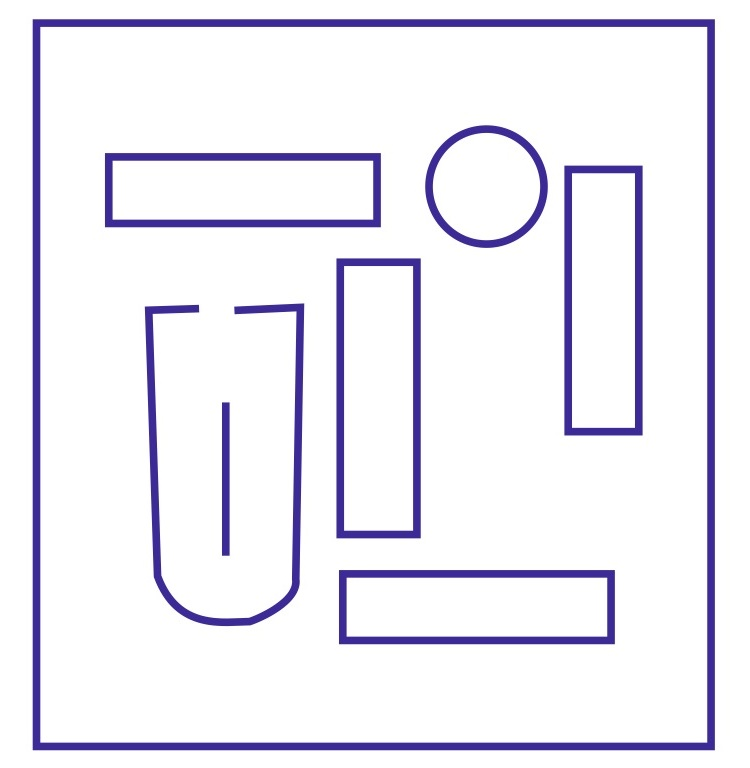Answer figure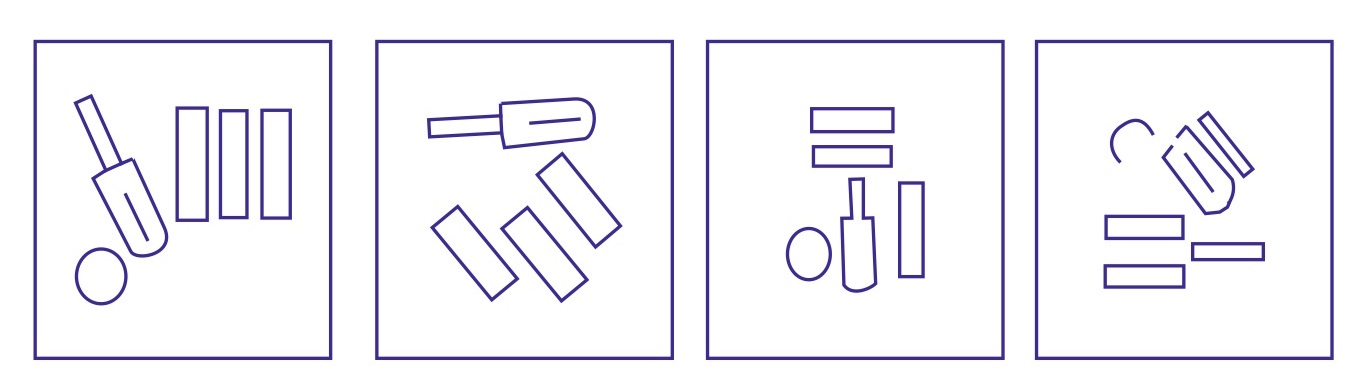(A)                                   (B)                               (C)                       (D)

Ans .   A

Q.2. Find the figure which includes the question figure.

Question figure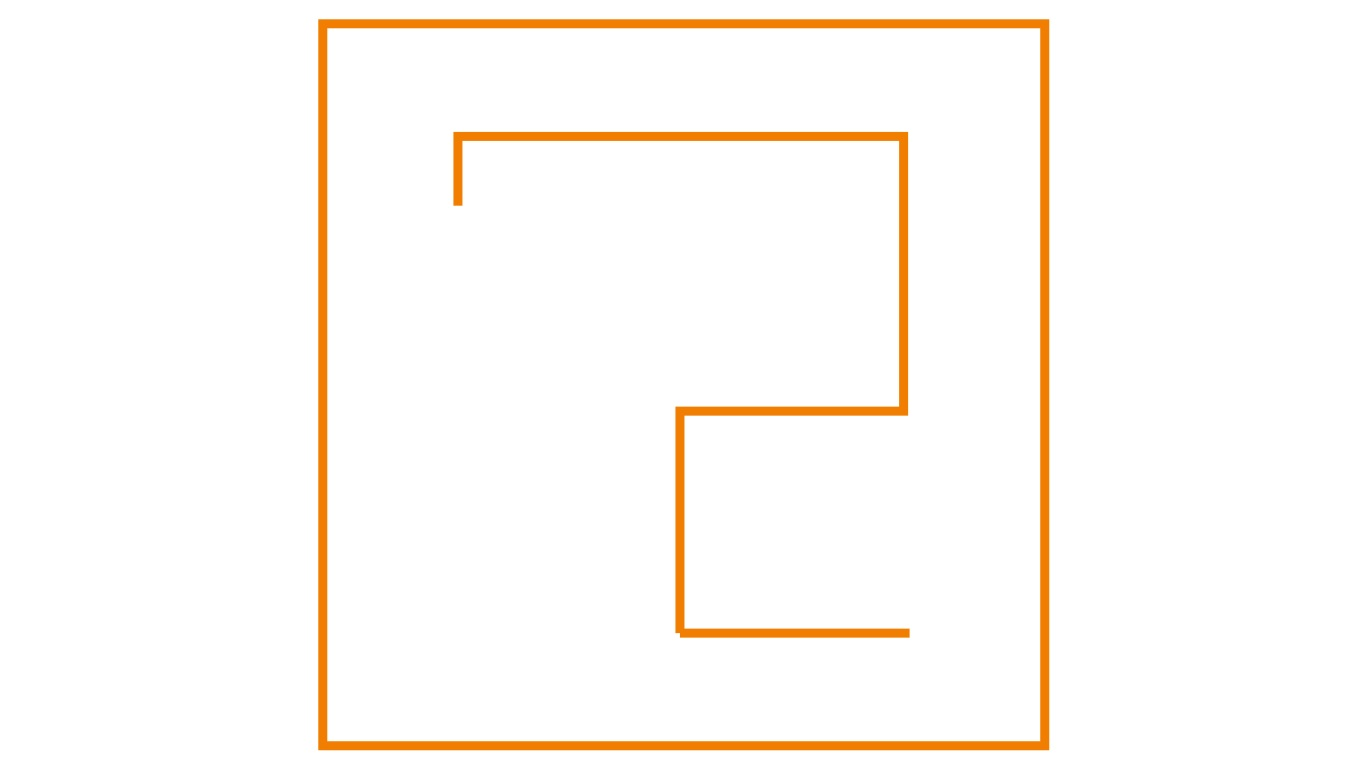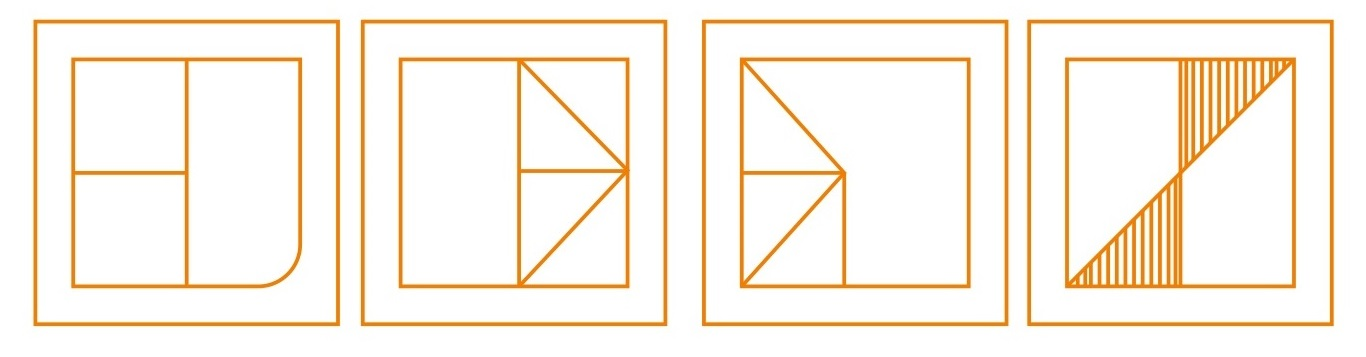(A)                                       (B)                                (C)                          (D)

Ans .   B

Q.3. Which of the answer figure in the following includes the question figure?

Question figure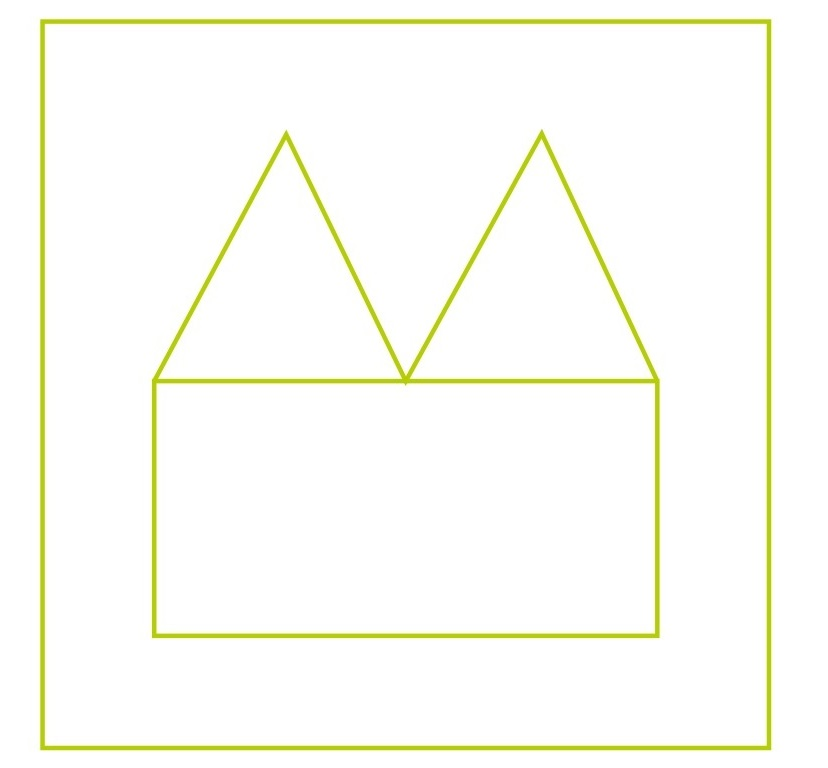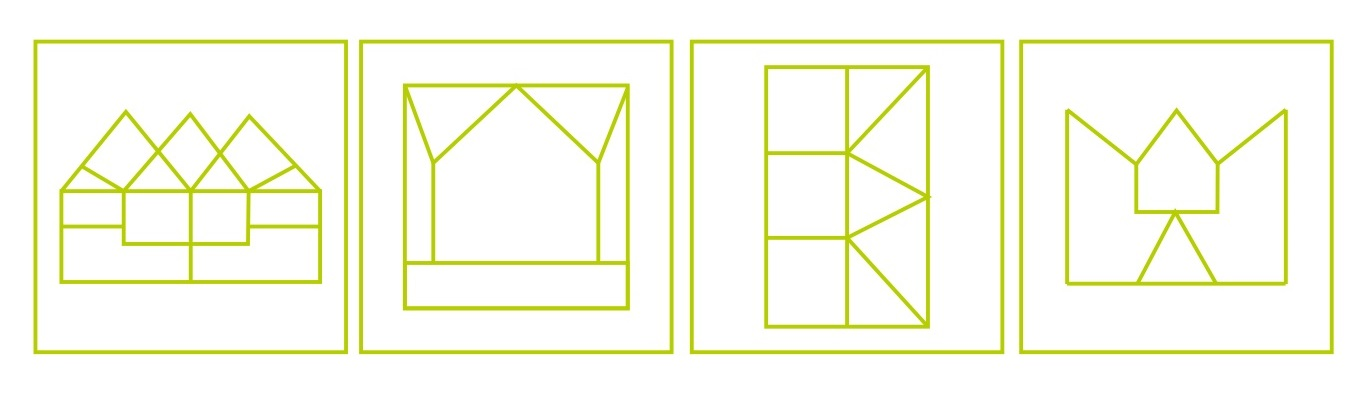(A)                              (B)                              (C)                      (D)

Ans .   A

Q.4.Find the answer figure which includes the question figure.

Question figure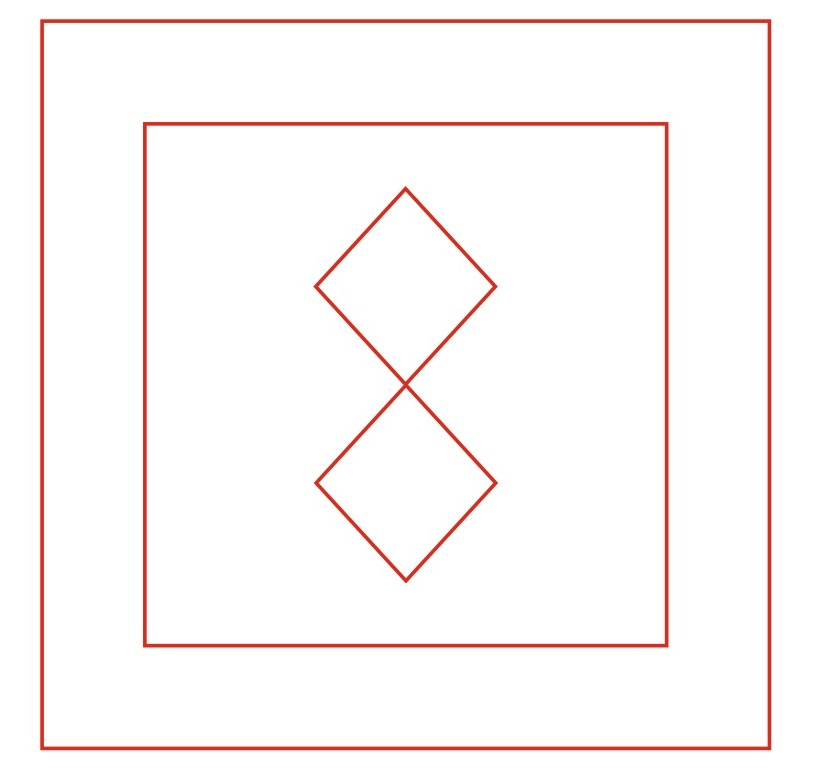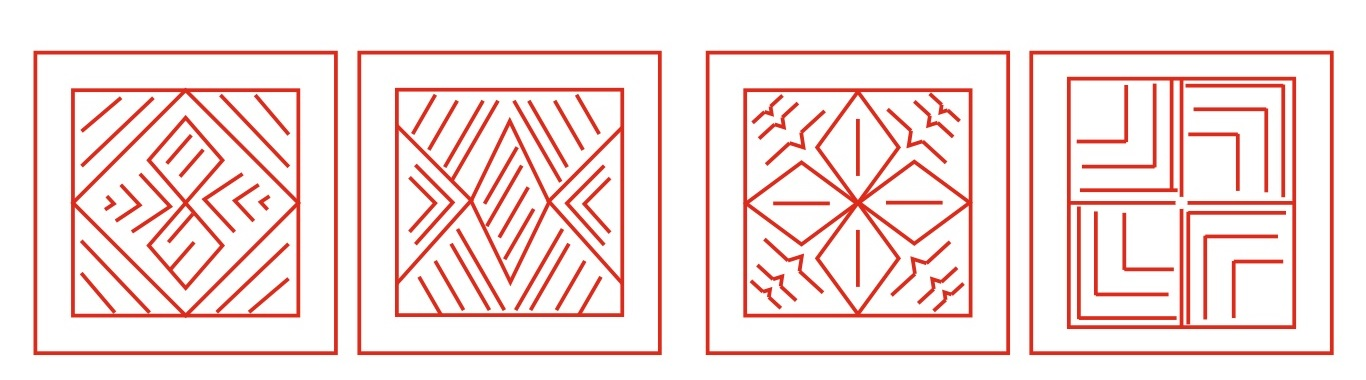(A)                                   (B)                                (C)                               (D)

Ans .   A

Q.5. Find the answer figure which includes the question figure.

Question figure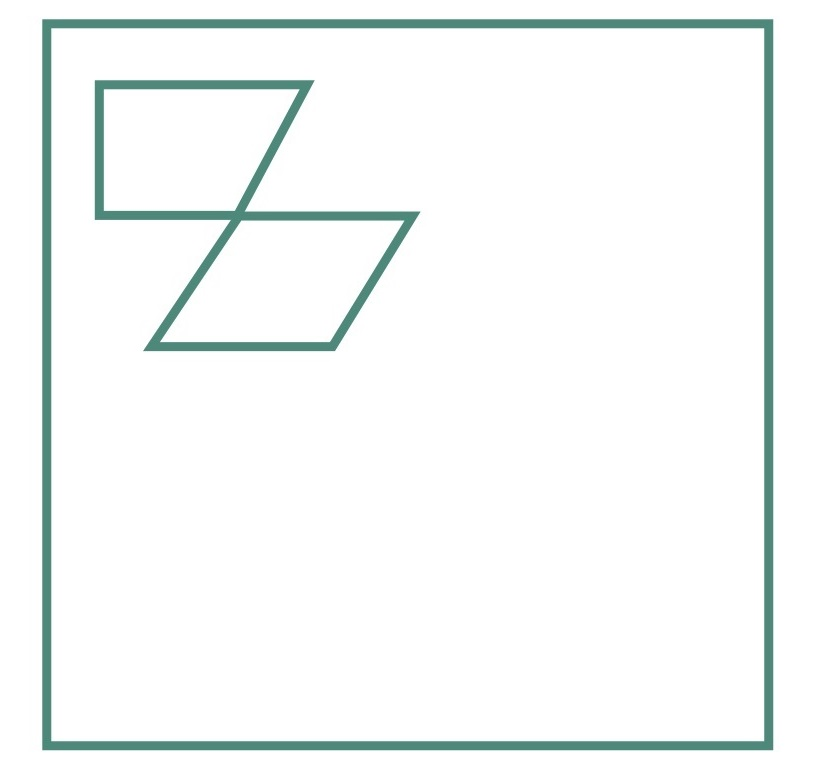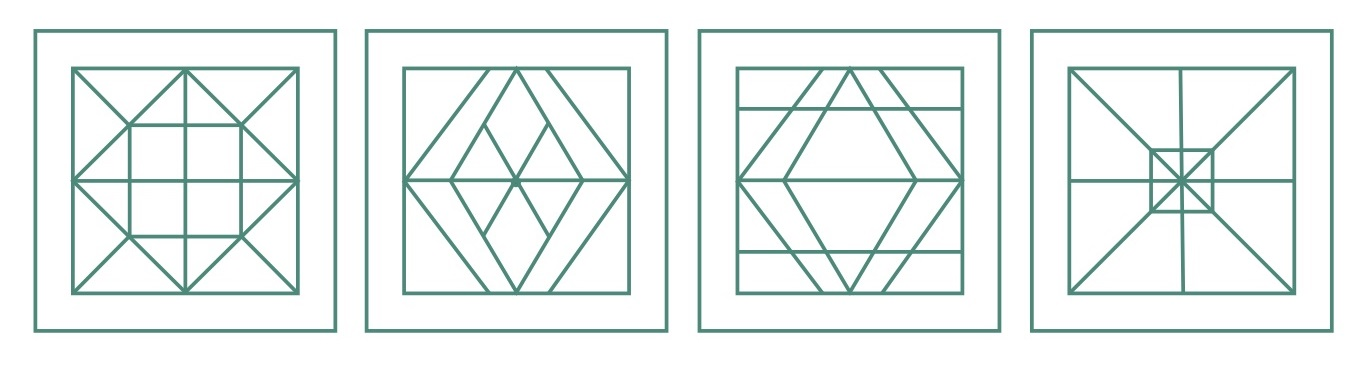(A)                                 (B)                           (C)                           (D)

Ans .   C

Q.6. In which answer figure, the question figure is included?

Question figure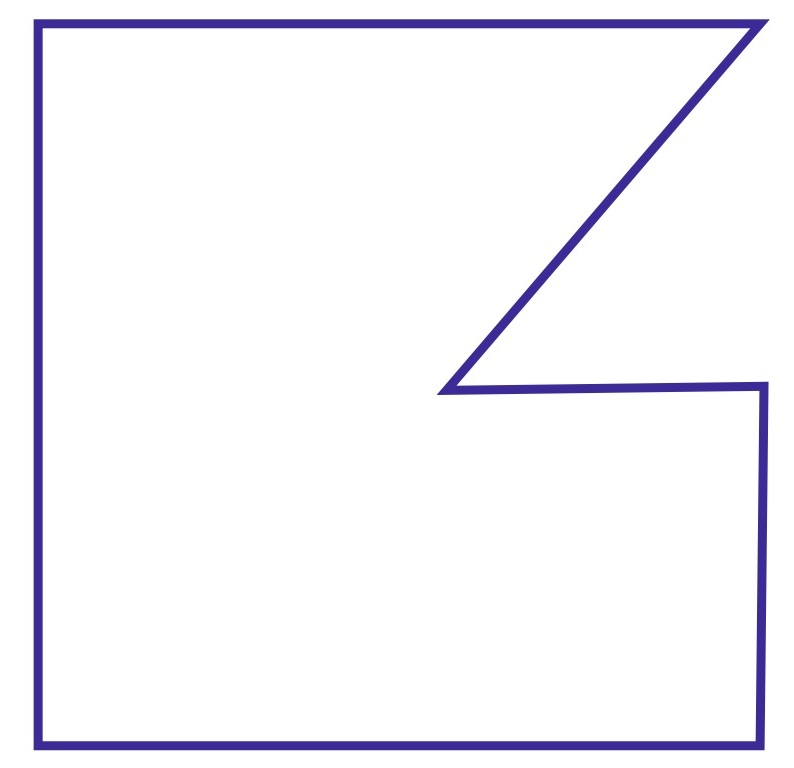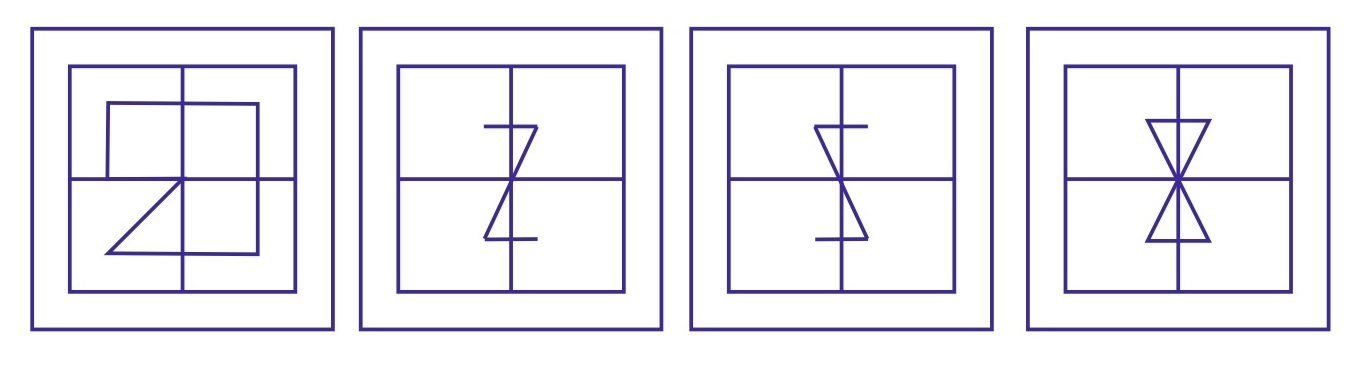(A)                                     (B)                            (C)                        (D)